JEE  >  VITEEE PCME Mock Test - 10

# VITEEE PCME Mock Test - 10

Test Description

## 125 Questions MCQ Test VITEEE: Subject Wise and Full Length MOCK Tests | VITEEE PCME Mock Test - 10

VITEEE PCME Mock Test - 10 for JEE 2023 is part of VITEEE: Subject Wise and Full Length MOCK Tests preparation. The VITEEE PCME Mock Test - 10 questions and answers have been prepared according to the JEE exam syllabus.The VITEEE PCME Mock Test - 10 MCQs are made for JEE 2023 Exam. Find important definitions, questions, notes, meanings, examples, exercises, MCQs and online tests for VITEEE PCME Mock Test - 10 below.
Solutions of VITEEE PCME Mock Test - 10 questions in English are available as part of our VITEEE: Subject Wise and Full Length MOCK Tests for JEE & VITEEE PCME Mock Test - 10 solutions in Hindi for VITEEE: Subject Wise and Full Length MOCK Tests course. Download more important topics, notes, lectures and mock test series for JEE Exam by signing up for free. Attempt VITEEE PCME Mock Test - 10 | 125 questions in 150 minutes | Mock test for JEE preparation | Free important questions MCQ to study VITEEE: Subject Wise and Full Length MOCK Tests for JEE Exam | Download free PDF with solutions
 1 Crore+ students have signed up on EduRev. Have you?
VITEEE PCME Mock Test - 10 - Question 1

### The maximum value of logx/x in (2, ∞) is

VITEEE PCME Mock Test - 10 - Question 2

### The area in square units of the region bounded by the curves x2 = 4y, the line x = 2 and the x-axis is

Detailed Solution for VITEEE PCME Mock Test - 10 - Question 2

The required area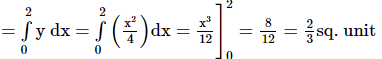VITEEE PCME Mock Test - 10 - Question 3

### The polar form of the complex number (i25 )3 is

VITEEE PCME Mock Test - 10 - Question 4

If [(1+i)/(1-i)]m = 1, the least value of m is

VITEEE PCME Mock Test - 10 - Question 5

The area bounded by two curves y2=4ax and x2=4ay is

VITEEE PCME Mock Test - 10 - Question 6

Let [ ] denote the greatest integer function and f(x) = [tan2x]. Then

VITEEE PCME Mock Test - 10 - Question 7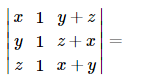VITEEE PCME Mock Test - 10 - Question 8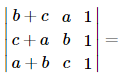VITEEE PCME Mock Test - 10 - Question 9

Consider f (x)   = x2/| x | , x ≠ 0, f(x)   = 0, x = 0

VITEEE PCME Mock Test - 10 - Question 10

The differential equation of the family of curves y2=4a (x+a) is

VITEEE PCME Mock Test - 10 - Question 11

The solution of the equation (1+x2)(1+y)dy+(1+x)(1+y2)dx=0 is

VITEEE PCME Mock Test - 10 - Question 12

Which equation has the solution, y = 4sin3x?

VITEEE PCME Mock Test - 10 - Question 13

Let A = { x ∈ R : x ≠ 0, − 4 ≤ x ≤ 4 } and f : A → R is defined by f (x)   = | x |/x for x ∈ A . Then the range of f is

VITEEE PCME Mock Test - 10 - Question 14

The solution of (x + y + 1)  dy/dx = 1 is

Detailed Solution for VITEEE PCME Mock Test - 10 - Question 14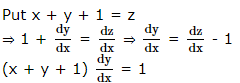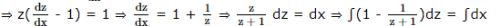⇒ z - log(z + 1) = x + c ⇒ x + y + 1 = log(x + y + 2) + x + c ⇒ y = log(x + y + 2) + log c
⇒ ey = (x + y + z)c ⇒ x + y + 2 = cey

VITEEE PCME Mock Test - 10 - Question 15

∫tan-1[(2x)/(1-x2)]dx=

VITEEE PCME Mock Test - 10 - Question 16

The derivative of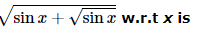VITEEE PCME Mock Test - 10 - Question 17

∫[(3x3-2√x)/(x)] dx =

VITEEE PCME Mock Test - 10 - Question 18

tan[(1/2)cos-1(√5/3)]=

VITEEE PCME Mock Test - 10 - Question 19

What is the value of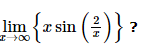VITEEE PCME Mock Test - 10 - Question 20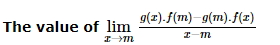if f(m) = 3, f'(m) = 2, g(m) = - 1 and g'(m) = 2 is

VITEEE PCME Mock Test - 10 - Question 21

Which of the following is not a statement ?

VITEEE PCME Mock Test - 10 - Question 22

All diagonal elements of skew symmetric matrix are

VITEEE PCME Mock Test - 10 - Question 23

The number of ways in which a committee of 6 members can be formed from 8 gentlemen and 4 ladies so that the committee contains atleast 3 ladies is

Detailed Solution for VITEEE PCME Mock Test - 10 - Question 23

A committee of 6 members is to formed from 8 gentlemen and 4 ladies by taking
(i) 3 lady out of 4 and 3 gentlemen out of 8
(ii) 4 lady out of 4 and 2 gentlemen out of 8
In case (i) the number of ways = 4c3 x 8c3
= 4 x 56 = 224
In case (ii) the number of ways = 4c4 x 8c2
= 1 x 28 = 28
Hence, the required number of ways = 224 + 28 = 252

VITEEE PCME Mock Test - 10 - Question 24

If three distinct numbers are chosen randomly from the first 100 natural numbers, then the probability that all three of them are divisible by both 2 and 3, is

VITEEE PCME Mock Test - 10 - Question 25

If A and B are square matrices of the same order, then

VITEEE PCME Mock Test - 10 - Question 26

If A and B are two events such that P(A) = p, P(B) = q, P (A ∩ B) = r , then the probability that A occurs but B does not occur, is

VITEEE PCME Mock Test - 10 - Question 27

If A and B are two independent events in a given sample space and the probability that both A and B occur is 0.16 while the probability that neither occurs is 0.36. The probabilities P(A) and P(B) are respectively

VITEEE PCME Mock Test - 10 - Question 28

In Δ A B C , If a2 + b2  + c2  = 8 R2 , then the triangle is

VITEEE PCME Mock Test - 10 - Question 29

If b + c, c + a, a + b are in H.P., then a2, b2, c2 will be in

VITEEE PCME Mock Test - 10 - Question 30

Mode is approximately given by

VITEEE PCME Mock Test - 10 - Question 31

The S.D. of 5 scores 1,2,3,4,5 is

VITEEE PCME Mock Test - 10 - Question 32

The condition for the roots of the equation (c2-ab)x2-2(a2-bc)x+(b2-ac)=d are equal, is

VITEEE PCME Mock Test - 10 - Question 33

If α,β are the roots of equation x+1=λx(1-λx) and two values of λ can be determined from the equation (α/β)+(β/α)=π-2, then (λ₁2/λ₂2)+(λ₂2/λ₁2)+2=

VITEEE PCME Mock Test - 10 - Question 34

The angle between the line (x + 1)/3 = (y - 1)/2 = (z - 2)/4 and the plane 2x + y - 3z + 4 = 0 is

VITEEE PCME Mock Test - 10 - Question 35

The general value of θ which satisfies tanθ=-1 and cosθ=(1/√2) the equations is

VITEEE PCME Mock Test - 10 - Question 36

The slope of the tangent at the point (h, h) of the circle x2 + y2 = ais

VITEEE PCME Mock Test - 10 - Question 37

The distance of the point (2, 1, -1) from the plane x - 2y + 4z = 9 is

VITEEE PCME Mock Test - 10 - Question 38

The equation y2 - x2 + 2x - 1 = 0 represents

VITEEE PCME Mock Test - 10 - Question 39

The angle between the vectors 3i+j+2k and 2i-2j+4k is

VITEEE PCME Mock Test - 10 - Question 40

If |a+b|=|a-b|, then the angle between a and b is

VITEEE PCME Mock Test - 10 - Question 41
An ideal transformer has 500 and 5000 turns in primary and secondary windings respectively. If the primary voltage is connected to a 6V battery then the secondary voltage is
VITEEE PCME Mock Test - 10 - Question 42
X - rays are
VITEEE PCME Mock Test - 10 - Question 43
To a charged particle, which is moving with a constant initial velocity $\stackrel{\to }{V}$, a uniform magnetic field is applied in the direction of velocity; then
VITEEE PCME Mock Test - 10 - Question 44
A parallel plate capacitor is charged. If the plates are pulled apart
VITEEE PCME Mock Test - 10 - Question 45

In short wave communication waves of which of the following frequencies will be reflected back by the ionospheric layer having electron density 1011 per m-3?

Detailed Solution for VITEEE PCME Mock Test - 10 - Question 45

N max = 1011 per m-3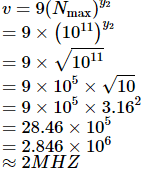VITEEE PCME Mock Test - 10 - Question 46
The masses of three wires of copper are in ratio 1 : 2 : 3 and their length are in the ratio 3 : 2 : 1. The ratio of their resistances is
VITEEE PCME Mock Test - 10 - Question 47
If 96500 C of electricity liberates one gram equivalent of any substance, the time taken for a current of 0.15 A to deposit 20 mg of copper from a solution of copper sulphate is (chemical equivalent of copper = 32)
VITEEE PCME Mock Test - 10 - Question 48
A microammeter has a resistance of 100 Ω and full scale range of 50 μA. It can be used as a voltmeter or as a higher range ammeter provided a resistance is added to it. Pick the correct range and resistance combination
VITEEE PCME Mock Test - 10 - Question 49
When a wire of resistance R is stretched and its radius becomes r/2, then the new resistance will be
VITEEE PCME Mock Test - 10 - Question 50
The resistance of a galvanometer coil is R. What is the shunt resistance required to convert it into an ammeter of range 4 times
VITEEE PCME Mock Test - 10 - Question 51
Photoelectric effect proves the
VITEEE PCME Mock Test - 10 - Question 52
What is self inductance of a which produces 5 V, when current in it changes from 3 A to 2 A in one millisecond ?
VITEEE PCME Mock Test - 10 - Question 53

The core of a transformer is laminated so that

VITEEE PCME Mock Test - 10 - Question 54

A step up transformer operates on a 230 volt line and supplies 2 amp to a load. The ratio of primary and secondary windings is 1:25. Determine the primary current .

Detailed Solution for VITEEE PCME Mock Test - 10 - Question 54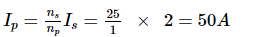VITEEE PCME Mock Test - 10 - Question 55
An accelerated electron would produce
VITEEE PCME Mock Test - 10 - Question 56
A charge q is placed at the corner of a cube of side l. The electric flux passing through the cube is
Detailed Solution for VITEEE PCME Mock Test - 10 - Question 56
Eight cubes, each side l, are required to form a Gaussian surface
So that the charge q at the corner of a small cube appears at the centre of the bigger cube
According to Gauss's law, the electric flux through the bigger cubes = $\frac{{q}_{}}{{\in }_{0}}$
Hence the electric flux through the given small cube = $\frac{{q}_{}}{{\mathrm{8\in }}_{0}}$
VITEEE PCME Mock Test - 10 - Question 57
Two charges -10 C and + 10 C are placed 10cm apart. Potential at the centre of the line joining two charges is
VITEEE PCME Mock Test - 10 - Question 58

Two equal charges q of opposite sign separated by a distance 2a constitute an electric dipole of dipole moment p. If P is a point at a distance r from the centre of the dipole and the line joining centre to this point makes an angle θ with the axis of the dipole, then potential at the point P is given by

VITEEE PCME Mock Test - 10 - Question 59
Figure shows an electric line of force which curves along a circular arc. The magnitude of electric field intensity is same at all points on this curve and is equal to E. If the potential at A is V, then the potential at B is:
Detailed Solution for VITEEE PCME Mock Test - 10 - Question 59 V = - ∫ E - dr
⇒ VB - VA = - ERθ
VB = V - ERθ
VITEEE PCME Mock Test - 10 - Question 60
At a point A, there is an electric field of 500 V-m⁻� and potential difference of 3000 V. The distance between the point charge and the point A is
VITEEE PCME Mock Test - 10 - Question 61

Two charges 'Q' and 'Q' are kept at a distance 'd' .The charge 'q' is kept at the midpoint of line joining the charges. The magnitude of 'q' to keep the system in equilibrium is

VITEEE PCME Mock Test - 10 - Question 62
A straight wire of diameter 0.5 mm carrying a current of 1 A is replaced by another wire of 1 mm diameter carrying the same current. The strength of magnetic field far away is
VITEEE PCME Mock Test - 10 - Question 63
The function of the moderator in a nuclear reactor is
VITEEE PCME Mock Test - 10 - Question 64
In a fission of U235 nucleus on average ----- neutrons are released.
Detailed Solution for VITEEE PCME Mock Test - 10 - Question 64 When U235 is bombarded by a neutron, it splits into barium,
Krypton and 2 or 3, so average 2.5 neutrons per fission
VITEEE PCME Mock Test - 10 - Question 65
A heavy nucleus at rest breaks into two fragments which fly off with velocities 8:1. The ratio of radii of the fragments is
VITEEE PCME Mock Test - 10 - Question 66
The minimum energy required to remove an electron is called
VITEEE PCME Mock Test - 10 - Question 67
The maximum energy received by the earth from the sun is in the form of
VITEEE PCME Mock Test - 10 - Question 68
The threshold wavelength is 2000 Å. The work function is
VITEEE PCME Mock Test - 10 - Question 69
The eye-piece used in biological microscope is
VITEEE PCME Mock Test - 10 - Question 70
In an n-p-n transistor, p-acts as a/an
VITEEE PCME Mock Test - 10 - Question 71

Pick out the incorrect statement regarding reverse saturation current in a p - n junction diode.

VITEEE PCME Mock Test - 10 - Question 72
In an intrinsic - semiconductor the charge carriers responsible for electrical conduction are
VITEEE PCME Mock Test - 10 - Question 73
In a P-type semiconductor, germanium is doped with
VITEEE PCME Mock Test - 10 - Question 74
The most commonly used material for making transistor is
VITEEE PCME Mock Test - 10 - Question 75
When a semiconductor is continuously heated the value of its resistance
VITEEE PCME Mock Test - 10 - Question 76
In N-type semiconductors, majority charge carriers are
VITEEE PCME Mock Test - 10 - Question 77
In a biprism expt., by using light of wavelength 5000 Å, 5 mm wide fringes are obtained on a screen 1.0 m away from coherent sources. The separation between two coherent sources is
VITEEE PCME Mock Test - 10 - Question 78

For the constructive interference the path difference between the two interfering waves must be equal to

VITEEE PCME Mock Test - 10 - Question 79
A train is approaching a platform with a velocity ν. The frequency heard by an observer standing at the station is
VITEEE PCME Mock Test - 10 - Question 80

In the propagation of light waves, the angle between the direction of vibration and plane of polarisation is

VITEEE PCME Mock Test - 10 - Question 81

Final product formed on reduction of glycerol by hydriodic acid is

VITEEE PCME Mock Test - 10 - Question 82

Two moles of an aldehyde react with concentrated solution of sodium hydroxide and produce one mole of acid and one mole of alcohol. The aldehyde is

VITEEE PCME Mock Test - 10 - Question 83

In Cannizzaro's reaction

VITEEE PCME Mock Test - 10 - Question 84

Which of the following method is most appropriate for the manufacture of methane?

VITEEE PCME Mock Test - 10 - Question 85

Formalin is an aqueous solution of

VITEEE PCME Mock Test - 10 - Question 86

Propene is reacted with HBr in presence of peroxide, the product is

VITEEE PCME Mock Test - 10 - Question 87

Which one of the following compounds is not a vitamin?

VITEEE PCME Mock Test - 10 - Question 88

Sodium ethoxide reacts with ethyl acetate to

VITEEE PCME Mock Test - 10 - Question 89

4.4 g of an unknown gas occupies 2.24 L of volume at standard temperature and pressure. The gas may be

VITEEE PCME Mock Test - 10 - Question 90

Scurvy is caused due to deficiency of

VITEEE PCME Mock Test - 10 - Question 91

The reagent which forms crystalline osazone derivative when reacted with glucose is

VITEEE PCME Mock Test - 10 - Question 92

The mass of 112 cm3 of CH₄ gas at STP is

VITEEE PCME Mock Test - 10 - Question 93

A cylinder filled with a movable piston contains liquid water in equilibrium with water vapour at 250C. Which one of the following operations results in a decreace in the equilibrium vapour pressure ?

VITEEE PCME Mock Test - 10 - Question 94

The enthalpy of a solution of KNO3 is + 35.64 kJ. This denotes

VITEEE PCME Mock Test - 10 - Question 95

Hydrolysis of benzonitrite by dilute HCl yields

VITEEE PCME Mock Test - 10 - Question 96

Hess's law deals with

VITEEE PCME Mock Test - 10 - Question 97

The acid showing salt like character in aqueous solutions is

VITEEE PCME Mock Test - 10 - Question 98

In a reversible reaction, the catalyst

VITEEE PCME Mock Test - 10 - Question 99

VITEEE PCME Mock Test - 10 - Question 100

In the presence of acid, the hydrolysis of methyl cyanide gives

VITEEE PCME Mock Test - 10 - Question 101

The d-electron configurations of Cr2+, Mn2+, Fe2+ and Ni2+ are 3d4, 3d5, 3d6 and 3d8 respectively. Which one of the following aqua complexes will exhibit the minimum paramagnetic behaviour?
(At No. Cr = 24, Mn = 25, Fe = 26, Ni = 28)

VITEEE PCME Mock Test - 10 - Question 102

The ingredient used as vehicle in perfumery is

VITEEE PCME Mock Test - 10 - Question 103

Copper sulphate dissolves in excess of KCN to give

VITEEE PCME Mock Test - 10 - Question 104

The IUPAC name of CH₃-C≡C-CH(CH₃)₂ is

VITEEE PCME Mock Test - 10 - Question 105

Which of the following reagent can be used to convert benzene diazonium chloride into benzene ?

VITEEE PCME Mock Test - 10 - Question 106

At STP,1.12 litre of H₂ is obtained on flowing a current for 965 seconds in a solution.The value of current is

VITEEE PCME Mock Test - 10 - Question 107

Which of the following reactions does not yield an alkyl halide?

VITEEE PCME Mock Test - 10 - Question 108

On heating quick lime with coke in an electric furnace, we get

VITEEE PCME Mock Test - 10 - Question 109

The single electrode reactions Cd(s) → Cd + 2(aq) + 2e- E0 = 0.403 V
Cu+2 (aq) + 2 e- → Cus E0  = 0.337 V can be combined to give the cell reaction
. Cd (s)   → Cu+2 (aq)    → Cd+2 (aq) + Cu s . The emf of such cell is

VITEEE PCME Mock Test - 10 - Question 110

In which of the following solutions are ions present?

VITEEE PCME Mock Test - 10 - Question 111

When the product of pressure and volume is plotted against pressure for a given amount of the gas, the line obtained is

VITEEE PCME Mock Test - 10 - Question 112

Which of the following halides is the most stable?

VITEEE PCME Mock Test - 10 - Question 113

Among the trihalides of nitrogen which one is most basic?

VITEEE PCME Mock Test - 10 - Question 114

The intermetallic compound LiAg crystallizes in cubic lattice in which both lithium and silver have co-ordination number of eight. The crystal class is

VITEEE PCME Mock Test - 10 - Question 115

A compound contains atoms of three elements : A,B and C. If the oxidation number of A is + 2, B is + 5 and that of C is -2, the possible formula of the compound is

VITEEE PCME Mock Test - 10 - Question 116

The oxide which is the anhydride of orthophosphoric acid is

VITEEE PCME Mock Test - 10 - Question 117

The bleeding on a wound is stopped by the application of ferric chloride due to

VITEEE PCME Mock Test - 10 - Question 118

In the ground state, an element has 13 electrons in its "M-shell". The element is.......

VITEEE PCME Mock Test - 10 - Question 119

The valence shell of transition elements consist of

VITEEE PCME Mock Test - 10 - Question 120

C4H4O4 can be:

VITEEE PCME Mock Test - 10 - Question 121

Find the Antonym of GATHERED

VITEEE PCME Mock Test - 10 - Question 122

Fill in the blank with appropriate word.
.........learning does not help the mental growth of a student.

VITEEE PCME Mock Test - 10 - Question 123

Find the synonym of ATTRIBUTE

VITEEE PCME Mock Test - 10 - Question 124

Choose the alternative which best expresses the meaning of the idiom/phrase.

Bring forth

VITEEE PCME Mock Test - 10 - Question 125

Improve the sentence by choosing best alternative for capitalised part of the sentence.

I waited for half an hour for my friend, but the train didn't TURN IN.

## VITEEE: Subject Wise and Full Length MOCK Tests

1 videos|3 docs|73 tests
 Use Code STAYHOME200 and get INR 200 additional OFF Use Coupon Code
Information about VITEEE PCME Mock Test - 10 Page
In this test you can find the Exam questions for VITEEE PCME Mock Test - 10 solved & explained in the simplest way possible. Besides giving Questions and answers for VITEEE PCME Mock Test - 10, EduRev gives you an ample number of Online tests for practice

## VITEEE: Subject Wise and Full Length MOCK Tests

1 videos|3 docs|73 tests